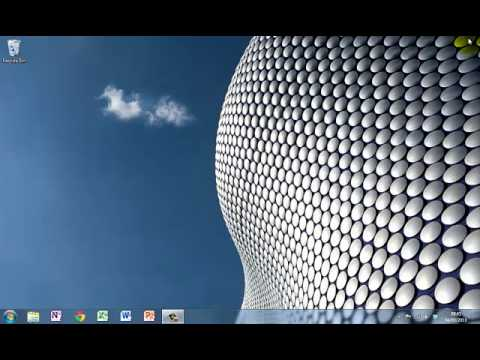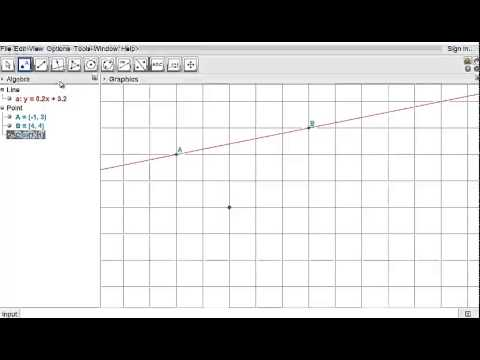﻿ Project Maths | ICT in the Classroom
Working together to improve teaching & learning Leagan Gaeilge
Professional Development ›› ICT in the Classroom

We have lots of tutorials full of helpful tips on using ICT effectively in your classroom.

### Learn to use GeoGebra

Want to know how to get started with GeoGebra? Want to find out how to integrate GeoGebra into your lessons? Want to learn to use GeoGebra for teaching functions, statistics or geometry? You’ll find lots of useful video tutorials and instructional manuals here.

Download GeoGebraIntroduction to geometryKnowledge of a few basic tools makes it easy to teach Geometry using Geogebra. This ...

The equation of a circleUnderstanding the equation of a circle is so much easier when you can see the circle...

See more GeoGebra

### Learn to use Calculators

It’s important that students are proficient in using their calculator. You’ll find video tutorials on all things calculator – from finding the mean of a dataset to verifying your solution to an equation – here.

Download GeoGebraIntroduction to geometryKnowledge of a few basic tools makes it easy to teach Geometry using Geogebra. This ...

The equation of a circleUnderstanding the equation of a circle is so much easier when you can see the circle...

See more Calculators

### Learn to use Census@School

Census@School is an invaluable resource for teaching statistics. Learn how to collect your own students’ data or get data from a random sample of students in a different country here.

Using Census@SchoolWatch a teacher use Census@School to help students understand statistics.

See more Census@School

### Learn other useful ICT

Microsoft PowerPoint, Microsoft OneNote and Microsoft Mix can be easily used to make maths lessons more engaging and effective. Check out our tutorials, which show you how, here.

Download GeoGebraIntroduction to geometryThe equation of a circle